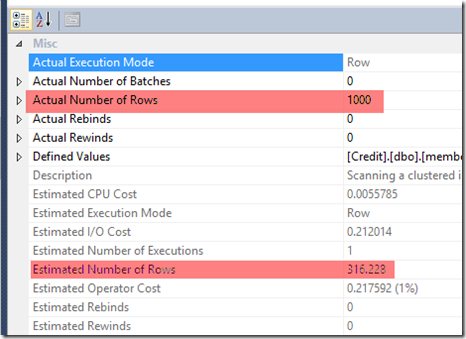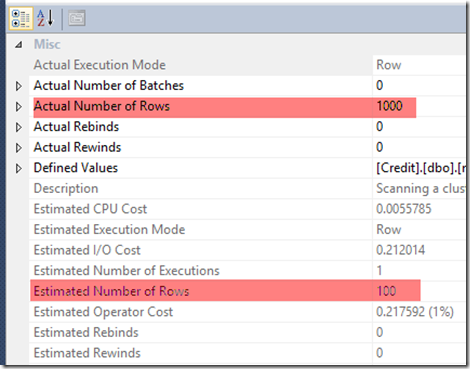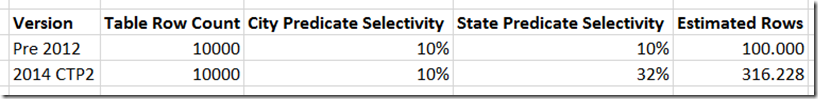# “CSelCalcCombineFilters_ExponentialBackoff” Calculator

One more post for the day, just while I have the blogging bug…

Per my last post, we saw that the query_optimizer_estimate_cardinality event can provide some interesting information in SQL Server 2014 around how cardinality estimates get calculated.

I have a setup I’ve used in the past to demonstrate column correlation challenges (see my post, Exploring Column Correlation and Cardinality Estimates, for the full example).  I’ll use that setup for this post as well, using the Credit database on SQL Server 2014 with the database compatibility level set to 120:

```USE [Credit];
GO

-- Modifying the Credit data to set up the DBA's scenario
UPDATE  [dbo].[member]
SET     [city] = 'Minneapolis',
[state_prov] = 'MN'
WHERE   [member_no] % 10 = 0;

UPDATE  [dbo].[member]
SET     [city] = 'New York',
[state_prov] = 'NY'
WHERE   [member_no] % 10 = 1;

UPDATE  [dbo].[member]
SET     [city] = 'Chicago',
[state_prov] = 'IL'
WHERE   [member_no] % 10 = 2;

UPDATE  [dbo].[member]
SET     [city] = 'Houston',
[state_prov] = 'TX'
WHERE   [member_no] % 10 = 3;

UPDATE  [dbo].[member]
[state_prov] = 'PA'
WHERE   [member_no] % 10 = 4;

UPDATE  [dbo].[member]
SET     [city] = 'Phoenix',
[state_prov] = 'AZ'
WHERE   [member_no] % 10 = 5;

UPDATE  [dbo].[member]
SET     [city] = 'San Antonio',
[state_prov] = 'TX'
WHERE   [member_no] % 10 = 6;

UPDATE  [dbo].[member]
SET     [city] = 'San Diego',
[state_prov] = 'CA'
WHERE   [member_no] % 10 = 7;

UPDATE  [dbo].[member]
SET     [city] = 'Dallas',
[state_prov] = 'TX'
WHERE   [member_no] % 10 = 8;
GO
```

So let’s look at the cardinality estimate for a specific operator in the following query (using RECOMPILE to get fresh query_optimizer_estimate_cardinality events on each execution):

```SELECT  m.[lastname],
m.[firstname],
SUM(c.[charge_amt]) AS [Total_Charge_amt]
FROM    [dbo].[member] AS [m]
INNER JOIN [dbo].[charge] AS  ON
m.[member_no] = c.[member_no]
WHERE   [city] = 'Minneapolis' AND
[state_prov] = 'MN'
GROUP BY m.[lastname],
m.[firstname]
OPTION (RECOMPILE);
GO
```

Below is the abridged plan and the operator and estimates I’m interested in:In SQL Server 2014 CTP2 – we estimate 316.228 rows for the Clustered Index Scan against the member table – and the actual number of rows is 1,000.

Now if I flip my database compatibility mode to pre-120, I’ll see the following actual vs. estimated instead:Before SQL Server 2014, the estimate for  “[city] = ‘Minneapolis’ AND  [state_prov] = ‘MN'” is 100 rows – and if we added the supporting statistics or index on city and state_prov, we’ll get a better estimate (but there are limitations to how useful this can be – another topic altogether, so see this post).  We know that, in this example, the two columns are correlated.  But we would need to help SQL Server if we didn’t want it to assume each predicate was independent.

Anyhow – the original issue pre SQL Server 2014 was that our city and state_prov columns were seen as independent, and so we saw our estimates reflect this (10% of row estimate for city multiplied by 10% of row estimate for state_prov).  So we wind up with an underestimate.

Now in SQL Server 2014 CTP2, with the Credit database compatibility mode put back to 120, we see the skew still exists, but the gap isn’t as extreme – showing 316.228 instead of 100 as an estimate.  Still incorrect, but less so.

Now putting this in Excel, we can see the following:Earlier we updated 10% of the 10,000 rows for various city/state combinations, so what’s up with the 32% for the state predicate selectivity?

That 32% is, using Excel formula style, =POWER((10%),1/2).

And if we look at the query_optimizer_estimate_cardinality for the 2014 CTP2 version, we’ll see the following calculator reference (abridged output):And here is the full calculator blurb for the CSelCalcCombineFilters_ExponentialBackoff:

<CalculatorList>
<FilterCalculator CalculatorName=”CSelCalcCombineFilters_ExponentialBackoff” Conjunction=”true”>
<SubCalculator>
<FilterCalculator CalculatorName=”CSelCalcColumnInInterval” Selectivity=”0.100″ TableName=”[m]” ColumnName=”state_prov” StatId=”5″ />
<FilterCalculator CalculatorName=”CSelCalcColumnInInterval” Selectivity=”0.100″ TableName=”[m]” ColumnName=”city” StatId=”4″ />
</SubCalculator>
</FilterCalculator>
</CalculatorList>

We see the selectivity of state_prov and city are both 0.100.  And yet unlike the pre-2014 version that multiples the two selectivity percentages, we’re not doing that in 2014 CTP2. The calculator name references “ExponentialBackoff” – which according to Wikipedia (yeah I know), is defined as “an algorithm that uses feedback to multiplicatively decrease the rate of some process, in order to gradually find an acceptable rate”.

What does this mean in this specific case?

In absence of multi-column statistics for city and state_prov, our estimated rows is not underestimated nearly as much as it used to be pre-2014.

Homework assignment – add another member column to the mix that has a 0.100 selectivity (for example – county) and compare the estimates using a pre-2014 and 2014 CTP2 compatibility mode.

## Other articles

### New Course: “SQL Server: Common Query Tuning Problems and Solutions – Part 2″

Today, Pluralsight published my latest course, “SQL Server: Common Query Tuning Problems and Solutions – Part 2“.  Part two is 2 hours and 17 minutes long

Explore

### New Course: “SQL Server: Common Query Tuning Problems and Solutions – Part 1”

Pluralsight published my latest course, “SQL Server: Common Query Tuning Problems and Solutions – Part 1“.  Part 1 is 2 hours and 31 minutes and

Explore

### SQLIntersection Post-Conference Session

I am really happy to announce that Kimberly Tripp and I will be delivering a post-conference in November’s SQLIntersection conference at the MGM Grand. The

Explore

### Optimizing Your Query Plans with the SQL Server 2014 Cardinality Estimator

I’ve been working on writing a new Microsoft white paper since January covering the main changes made in the SQL Server 2014 Cardinality Estimator, and

Explore

### Finding Timeout-Prone Code with max_elapsed_time

Consider a scenario where your application timeout setting is 30 seconds and you’ve been asked to proactively start tuning workloads that are at risk of

Explore

### Deck from “Practical SQL Server Cardinality Estimation”

I uploaded the deck from my session for SQLSaturday #287 Madison 2014 and you can download it here. It was an excellent event!  Great organizers,

Explore

## Imagine feeling confident enough to handle whatever your database throws at you.

With training and consulting from SQLskills, you’ll be able to solve big problems, elevate your team’s capacity, and take control of your data career.